Orals
Fan Wang · Oscar Madrid · Yi Yu · Alessandro Rinaldo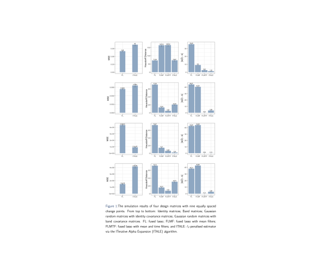We study the theoretical properties of the fused lasso procedure originally proposed by \cite{tibshirani2005sparsity} in the context of a linear regression model in which the regression coefficient are totally ordered and assumed to be sparse and piecewise constant. Despite its popularity, to the best of our knowledge, estimation error bounds in high-dimensional settings have only been obtained for the simple case in which the design matrix is the identity matrix. We formulate a novel restricted isometry condition on the design matrix that is tailored to the fused lasso estimator and derive estimation bounds for both the constrained version of the fused lasso assuming dense coefficients and for its penalised version. We observe that the estimation error can be dominated by either the lasso or the fused lasso rate, depending on whether the number of non-zero coefficient is larger than the number of piece-wise constant segments. Finally, we devise a post-processing procedure to recover the piecewise-constant pattern of the coefficients. Extensive numerical experiments support our theoretical findings.

Tianyi Liu · Yan Li · Enlu Zhou · Tuo Zhao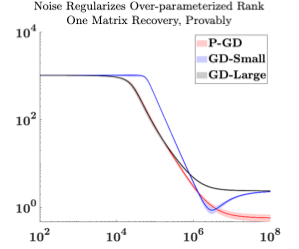We investigate the role of noise in optimization algorithms for learning over-parameterized models. Specifically, we consider the recovery of a rank one matrix $Y^*\in R^{d\times d}$ from a noisy observation $Y$ using an over-parameterization model. Specifically, we parameterize the rank one matrix $Y^*$ by $XX^\top$, where $X\in R^{d\times d}$. We then show that under mild conditions, the estimator, obtained by the randomly perturbed gradient descent algorithm using the square loss function, attains a mean square error of $O(\sigma^2/d)$, where $\sigma^2$ is the variance of the observational noise. In contrast, the estimator obtained by gradient descent without random perturbation only attains a mean square error of $O(\sigma^2)$. Our result partially justifies the implicit regularization effect of noise when learning over-parameterized models, and provides new understanding of training over-parameterized neural networks.
David Rindt · Robert Hu · David Steinsaltz · Dino Sejdinovic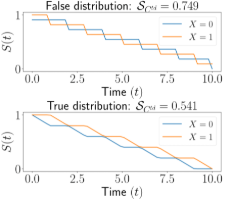We consider frequently used scoring rules for right-censored survival regression models such as time-dependent concordance, survival-CRPS, integrated Brier score and integrated binomial log-likelihood, and prove that neither of them is a proper scoring rule. This means that the true survival distribution may be scored worse than incorrect distributions, leading to inaccurate estimation. We prove, in contrast to these scores, that the right-censored log-likelihood is a proper scoring rule, i.e. the highest expected score is achieved by the true distribution. Despite this, modern feed-forward neural-network-based survival regression models are unable to train and validate directly on right-censored log-likelihood, due to its intractability, and resort to the aforementioned alternatives, i.e. non-proper scoring rules. We therefore propose a simple novel survival regression method capable of directly optimizing log-likelihood using a monotonic restriction on the time-dependent weights, coined SurvivalMonotonic-net (SuMo-net). SuMo-net achieves state-of-the-art log-likelihood scores across several datasets with 20--100x computational speedup on inference over existing state-of-the-art neural methods and is readily applicable to datasets with several million observations.

Kelvin Kan · François-Xavier Aubet · Tim Januschowski · Youngsuk Park · Konstantinos Benidis · Lars Ruthotto · Jan GasthausWe propose Multivariate Quantile Function Forecaster (MQF2), a global probabilistic forecasting method constructed using a multivariate quantile function and investigate its application to multi-horizon forecasting. Prior approaches are either autoregressive, implicitly capturing the dependency structure across time but exhibiting error accumulation with increasing forecast horizons, or multi-horizon sequence-to-sequence models, which do not exhibit error accumulation, but also do typically not model the dependency structure across time steps. MQF2 combines the benefits of both approaches, by directly making predictions in the form of a multivariate quantile function, defined as the gradient of a convex function which we parametrize using input-convex neural networks. By design, the quantile function is monotone with respect to the input quantile levels and hence avoids quantile crossing. We provide two options to train MQF2: with energy score or with maximum likelihood. Experimental results on real-world and synthetic datasets show that our model has comparable performance with state-of-the-art methods in terms of single time step metrics while capturing the time dependency structure.

Sanmitra Ghosh · Paul J. Birrell · Daniela De Angelis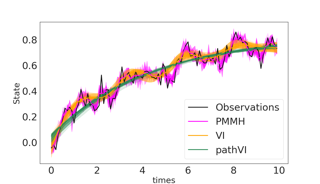By invoking a pathwise series expansion of Brownian motion, we propose to approximate a stochastic differential equation (SDE) with an ordinary differential equation (ODE). This allows us to reformulate Bayesian inference for a SDE as the parameter estimation task for an ODE. Unlike a nonlinear SDE, the likelihood for an ODE model is tractable and its gradient can be obtained using adjoint sensitivity analysis. This reformulation allows us to use an efficient sampler, such as NUTS, that rely on the gradient of the log posterior. Applying the reparameterisation trick, variational inference can also be used for the same estimation task. We illustrate the proposed method on a variety of SDE models. We obtain similar parameter estimates when compared to data augmentation techniques.

Masahiro Nakano · Ryo Nishikimi · Yasuhiro Fujiwara · Akisato Kimura · Takeshi Yamada · Naonori UedaThis paper addresses the question, ''What is the smallest object that contains all rectangular partitions with n or fewer blocks?'' and shows its application to relational data analysis using a new strategy we call super Bayes as an alternative to Bayesian nonparametric (BNP) methods. Conventionally, standard BNP methods have combined the Aldous-Hoover-Kallenberg representation with parsimonious stochastic processes on rectangular partitioning to construct BNP relational models. As a result, conventional methods face the great difficulty of searching for a parsimonious random rectangular partition that fits the observed data well in Bayesian inference. As a way to essentially avoid such a problem, we propose a strategy to combine an extremely redundant rectangular partition as a deterministic (non-probabilistic) object. Specifically, we introduce a special kind of rectangular partitioning, which we call superrectangulation, that contains all possible rectangular partitions. Delightfully, this strategy completely eliminates the difficult task of searching around for random rectangular partitions, since the superrectangulation is deterministically fixed in inference. Experiments on predictive performance in relational data analysis show that the super Bayesian model provides a more stable analysis than the existing BNP models, which are less likely to be trapped in bad local optima.

Charita Dellaporta · Jeremias Knoblauch · Theodoros Damoulas · Francois-Xavier Briol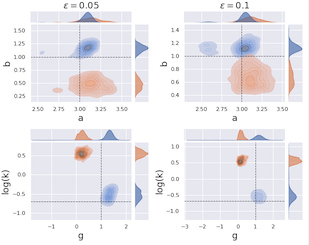Simulator-based models are models for which the likelihood is intractable but simulation of synthetic data is possible. They are often used to describe complex real-world phenomena, and as such can often be misspecified in practice. Unfortunately, existing Bayesian approaches for simulators are known to perform poorly in those cases. In this paper, we propose a novel algorithm based on the posterior bootstrap and maximum mean discrepancy estimators. This leads to a highly-parallelisable Bayesian inference algorithm with strong robustness properties. This is demonstrated through an in-depth theoretical study which includes generalisation bounds and proofs of frequentist consistency and robustness of our posterior. The approach is then assessed on a range of examples including a g-and-k distribution and a toggle-switch model.

Frederik BenzingContinual Learning addresses the challenge of learning a number of different tasks sequentially. The goal of maintaining knowledge of earlier tasks without re-accessing them starkly conflicts with standard SGD training for artificial neural networks. An influential method to tackle this problem without storing old data are so-called regularisation approaches. They measure the importance of each parameter for solving a given task and subsequently protect important parameters from large changes. In the literature, three ways to measure parameter importance have been put forward and they have inspired a large body of follow-up work. Here, we present strong theoretical and empirical evidence that these three methods, Elastic Weight Consolidation (EWC), Synaptic Intelligence (SI) and Memory Aware Synapses (MAS), are surprisingly similar and are all linked to the same theoretical quantity. Concretely, we show that, despite stemming from very different motivations, both SI and MAS approximate the square root of the Fisher Information, with the Fisher being the theoretically justified basis of EWC. Moreover, we show that for SI the relation to the Fisher -- and in fact its performance -- is due to a previously unknown bias. On top of uncovering unknown similarities and unifying regularisation approaches, we also demonstrate that our …

Vibhor Porwal · Piyush Srivastava · Gaurav Sinha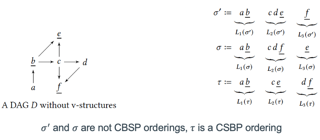A well-studied challenge that arises in the structure learning problem of causal directed acyclic graphs (DAG) is that using observational data, one can only learn the graph up to a "Markov equivalence class" (MEC). The remaining undirected edges have to be oriented using interventions, which can be very expensive to perform in applications. Thus, the problem of minimizing the number of interventions needed to fully orient the MEC has received a lot of recent attention, and is also the focus of this work. We prove two main results. The first is a new universal lower bound on the number of atomic interventions that any algorithm (whether active or passive) would need to perform in order to orient a given MEC. Our second result shows that this bound is, in fact, within a factor of two of the size of the smallest set of atomic interventions that can orient the MEC. Our lower bound is provably better than previously known lower bounds. The proof of our lower bound is based on the new notion of clique-block shared-parents (CBSP) orderings, which are topological orderings of DAGs without v-structures and satisfy certain special properties. Further, using simulations on synthetic graphs and by giving …

Guillaume Martinet · Alexander Strzalkowski · Barbara Engelhardt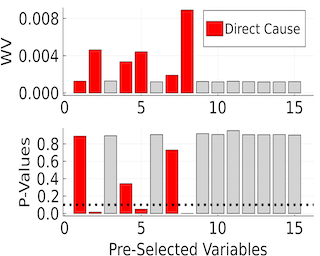Selecting powerful predictors for an outcome is a cornerstone task for machine learning. However, some types of questions can only be answered by identifying the predictors that causally affect the outcome. A recent approach to this causal inference problem leverages the invariance property of a causal mechanism across differing experimental environments (Peters et al., 2016; Heinze-Deml et al., 2018). This method, invariant causal prediction (ICP), has a substantial computational defect -- the runtime scales exponentially with the number of possible causal variables. In this work, we show that the approach taken in ICP may be reformulated as a series of nonparametric tests that scales linearly in the number of predictors. Each of these tests relies on the minimization of a novel loss function -- the Wasserstein variance -- that is derived from tools in optimal transport theory and is used to quantify distributional variability across environments. We prove under mild assumptions that our method is able to recover the set of identifiable direct causes, and we demonstrate in our experiments that it is competitive with other benchmark causal discovery algorithms.

Claudia Shi · Dhanya Sridhar · Vishal Misra · David BleiSynthetic control (SC) methods have been widely applied to estimate the causal effect of large-scale interventions, e.g., the state-wide effect of a change in policy.The idea of synthetic controls is to approximate one unit's counterfactual outcomes using a weighted combination of some other units' observed outcomes.The motivating question of this paper is: how does the SC strategy lead to valid causal inferences?We address this question by re-formulating the causal inference problem targeted by SC with a more fine-grained model, where we change the unit of analysis from large units" (e.g., states) tosmall units" (e.g., individuals in states).Under the re-formulation, we derive sufficient conditions for the non-parametric causal identification of the causal effect.We show that, in some settings, existing linear SC estimators are valid even when the data generating process is non-linear.We highlight two implications of the reformulation: 1) it clarifies where linearity" comes from, and how it falls naturally out of the more fine-grained and flexible model; 2) it suggests new ways of using available data with SC methods for valid causal inference, in particular, new ways of selecting observations from which to estimate the counterfactual.Given a graph, the densest subgraph problem asks for a set of vertices such that the average degree among these vertices is maximized. Densest subgraph has numerous applications in learning, e.g., community detection in social networks, link spam detection, correlation mining, bioinformatics, and so on. Although there are efficient algorithms that output either exact or approximate solutions to the densest subgraph problem, existing algorithms may violate the privacy of the individuals in the network, e.g., leaking the existence/non-existence of edges.In this paper, we study the densest subgraph problem in the framework of the differential privacy, and we derive the upper and lower bounds for this problem. We show that there exists a linear-time \epsilon-differentially private algorithm that finds a 2-approximation of the densest subgraph with an extra poly-logarithmic additive error. Our algorithm not only reports the approximate density of the densest subgraph, but also reports the vertices that form the densesubgraph.Our upper bound almost matches the famous 2-approximation by Charikar both in performance and in approximation ratio, but we additionally achieve differential privacy. In comparison with Charikar’s algorithm, our algorithm has an extra poly logarithmic additive error. We partly justify the additive error with a new lower bound, showing that …

Youming Tao · Yulian Wu · Peng Zhao · Di Wang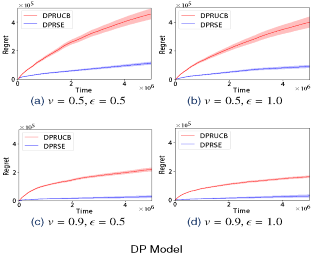In this paper we investigate the problem of stochastic multi-armed bandits (MAB) in the (local) differential privacy (DP/LDP) model. Unlike previous results that assume bounded/sub-Gaussian reward distributions, we focus on the setting where each arm's reward distribution only has $(1+v)$-th moment with some $v\in (0, 1]$. In the first part, we study the problem in the central $\epsilon$-DP model. We first provide a near-optimal result by developing a private and robust Upper Confidence Bound (UCB) algorithm. Then, we improve the result via a private and robust version of the Successive Elimination (SE) algorithm. Finally, we establish the lower bound to show that the instance-dependent regret of our improved algorithm is optimal. In the second part, we study the problem in the $\epsilon$-LDP model. We propose an algorithm that can be seen as locally private and robust version of SE algorithm, which provably achieves (near) optimal rates for both instance-dependent and instance-independent regret. Our results reveal differences between the problem of private MAB with bounded/sub-Gaussian rewards and heavy-tailed rewards. To achieve these (near) optimal rates, we develop several new hard instances and private robust estimators as byproducts, which might be used to other related problems. Finally, experiments also support our theoretical …
Dirk van der Hoeven · Nicolò Cesa-Bianchi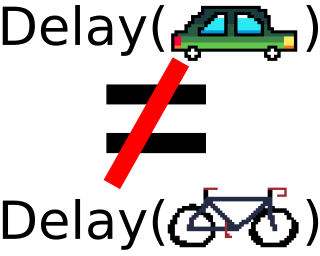We study nonstochastic bandits and experts in a delayed setting where delays depend on both time and arms. While the setting in which delays only depend on time has been extensively studied, the arm-dependent delay setting better captures real-world applications at the cost of introducing new technical challenges.In the full information (experts) setting, we design an algorithm with a first-order regret bound that reveals an interesting trade-off between delays and losses. We prove a similar first-order regret bound also for the bandit setting, when the learner is allowed to observe how many losses are missing.Our bounds are the first in the delayed setting that only depend on the losses and delays of the best arm.In the bandit setting, when no information other than the losses is observed, we still manage to prove a regret bound for bandits through a modification to the algorithm of \citet{zimmert2020optimal}.Our analyses hinge on a novel bound on the drift, measuring how much better an algorithm can perform when given a look-ahead of one round.

Jianyu Xu · Yu-Xiang WangIn feature-based dynamic pricing, a seller sets appropriate prices for a sequence of products (described by feature vectors) on the fly by learning from the binary outcomes of previous sales sessions ("Sold" if valuation $\geq$ price, and "Not Sold" otherwise). Existing works either assume noiseless linear valuation or precisely-known noise distribution, which limits the applicability of those algorithms in practice when these assumptions are hard to verify. In this work, we study two more agnostic models: (a) a "linear policy" problem where we aim at competing with the best linear pricing policy while making no assumptions on the data, and (b) a "linear noisy valuation" problem where the random valuation is linear plus an unknown and assumption-free noise. For the former model, we show a $\Theta(d^{1/3}T^{2/3})$ minimax regret up to logarithmic factors. For the latter model, we present an algorithm that achieves an $O(T^{3/4})$ regret and improve the best-known lower bound from $Omega(T^{3/5})$ to $\Omega(T^{2/3})$. These results demonstrate that no-regret learning is possible for feature-based dynamic pricing under weak assumptions, but also reveal a disappointing fact that the seemingly richer pricing feedback is not significantly more useful than the bandit-feedback in regret reduction.
Ted Moskovitz · Michael Arbel · Jack Parker-Holder · Aldo Pacchiano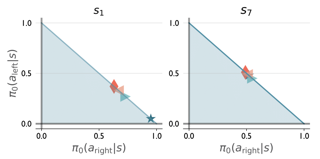Much of the recent success of deep reinforcement learning has been driven by regularized policy optimization (RPO) algorithms with strong performance across multiple domains. In this family of methods, agents are trained to maximize cumulative reward while penalizing deviation in behavior from some reference, or default policy. In addition to empirical success, there is a strong theoretical foundation for understanding RPO methods applied to single tasks, with connections to natural gradient, trust region, and variational approaches. However, there is limited formal understanding of desirable properties for default policies in the multitask setting, an increasingly important domain as the field shifts towards training more generally capable agents. Here, we take a first step towards filling this gap by formally linking the quality of the default policy to its effect on optimization. Using these results, we then derive a principled RPO algorithm for multitask learning with strong performance guarantees.

Jia-Jie Zhu · Christina Kouridi · Yassine Nemmour · Bernhard Schölkopf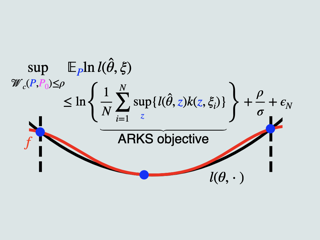We propose a scalable robust learning algorithm combining kernel smoothing and robust optimization. Our method is motivated by the convex analysis perspective of distributionally robust optimization based on probability metrics, such as the Wasserstein distance and the maximum mean discrepancy. We adapt the integral operator using supremal convolution in convex analysis to form a novel function majorant used for enforcing robustness. Our method is simple in form and applies to general loss functions and machine learning models. Exploiting a connection with optimal transport, we prove theoretical guarantees for certified robustness under distribution shift. Furthermore, we report experiments with general machine learning models, such as deep neural networks, to demonstrate competitive performance with the state-of-the-art certifiable robust learning algorithms based on the Wasserstein distance.

Tianyi Chen · Yuejiao Sun · Quan Xiao · Wotao Yin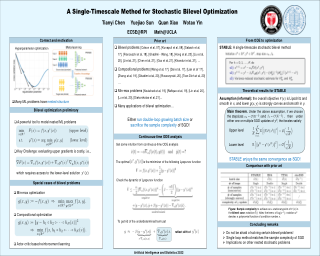Stochastic bilevel optimization generalizes the classic stochastic optimization from the minimization of a single objective to the minimization of an objective function that depends on the solution of another optimization problem. Recently, bilevel optimization is regaining popularity in emerging machine learning applications such as hyper-parameter optimization and model-agnostic meta learning. To solve this class of optimization problems, existing methods require either double-loop or two-timescale updates, which are sometimes less efficient. This paper develops a new optimization method for a class of stochastic bilevel problems that we term Single-Timescale stochAstic BiLevEl optimization (\textbf{STABLE}) method. STABLE runs in a single loop fashion, and uses a single-timescale update with a fixed batch size. To achieve an $\epsilon$-stationary point of the bilevel problem, STABLE requires ${\cal O}(\epsilon^{-2})$ samples in total; and to achieve an $\epsilon$-optimal solution in the strongly convex case, STABLE requires ${\cal O}(\epsilon^{-1})$ samples. To the best of our knowledge, when STABLE was proposed, it is the \emph{first} bilevel optimization algorithm achieving the same order of sample complexity as SGD for single-level stochastic optimization.
Kiran Thekumparampil · Niao He · Sewoong Oh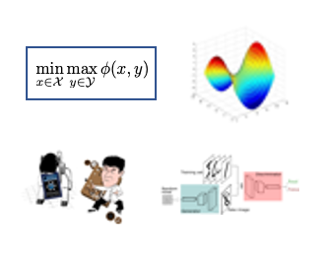We study the bilinearly coupled minimax problem: $\min_{x} \max_{y} f(x) + y^\top A x - h(y)$, where $f$ and $h$ are both strongly convex smooth functions and admit first-order gradient oracles. Surprisingly, no known first-order algorithms have hitherto achieved the lower complexity bound of $\Omega((\sqrt{\frac{L_x}{\mu_x}} + \frac{\|A\|}{\sqrt{\mu_x \mu_y}} + \sqrt{\frac{L_y}{\mu_y}}) \log(\frac1{\varepsilon}))$ for solving this problem up to an $\varepsilon$ primal-dual gap in the general parameter regime, where $L_x, L_y,\mu_x,\mu_y$ are the corresponding smoothness and strongly convexity constants. We close this gap by devising the first optimal algorithm, the Lifted Primal-Dual (LPD) method. Our method lifts the objective into an extended form that allows both the smooth terms and the bilinear term to be handled optimally and seamlessly with the same primal-dual framework. Besides optimality, our method yields a desirably simple single-loop algorithm that uses only one gradient oracle call per iteration. Moreover, when $f$ is just convex, the same algorithm applied to a smoothed objective achieves the nearly optimal iteration complexity. We also provide a direct single-loop algorithm, using the LPD method, that achieves the iteration complexity of $O(\sqrt{\frac{L_x}{\varepsilon}} + \frac{\|A\|}{\sqrt{\mu_y \varepsilon}} + \sqrt{\frac{L_y}{\varepsilon}})$. Numerical experiments on quadratic minimax problems and policy evaluation problems further demonstrate the fast convergence of …
Emilien Dupont · Yee Whye Teh · Arnaud Doucet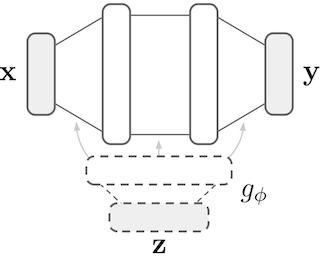Generative models are typically trained on grid-like data such as images. As a result, the size of these models usually scales directly with the underlying grid resolution. In this paper, we abandon discretized grids and instead parameterize individual data points by continuous functions. We then build generative models by learning distributions over such functions. By treating data points as functions, we can abstract away from the specific type of data we train on and construct models that are agnostic to discretization. To train our model, we use an adversarial approach with a discriminator that acts on continuous signals. Through experiments on a wide variety of data modalities including images, 3D shapes and climate data, we demonstrate that our model can learn rich distributions of functions independently of data type and resolution.

Saeid Naderiparizi · Adam Scibior · Andreas Munk · Mehrdad Ghadiri · Atilim Gunes Baydin · Bradley Gram-Hansen · Christian Schroeder de Witt · Robert Zinkov · Philip Torr · Tom Rainforth · Yee Whye Teh · Frank Wood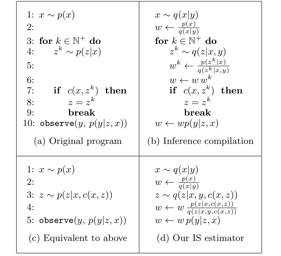Naive approaches to amortized inference in probabilistic programs with unbounded loops can produce estimators with infinite variance. This is particularly true of importance sampling inference in programs that explicitly include rejection sampling as part of the user-programmed generative procedure. In this paper we develop a new and efficient amortized importance sampling estimator. We prove finite variance of our estimator and empirically demonstrate our method's correctness and efficiency compared to existing alternatives on generative programs containing rejection sampling loops and discuss how to implement our method in a generic probabilistic programming framework.

Anna Korba · François PortierAdaptive importance sampling is a widely spread Monte Carlo technique that uses a re-weighting strategy to iteratively estimate the so-called target distribution. A major drawback of adaptive importance sampling is the large variance of the weights which is known to badly impact the accuracy of the estimates. This paper investigates a regularization strategy whose basic principle is to raise the importance weights at a certain power. This regularization parameter, that might evolve between zero and one during the algorithm, is shown (i) to balance between the bias and the variance and (ii) to be connected to the mirror descent framework. Using a kernel density estimate to build the sampling policy, the uniform convergence is established under mild conditions. Finally, several practical ways to choose the regularization parameter are discussed and the benefits of the proposed approach are illustrated empirically.

Oliver Richardson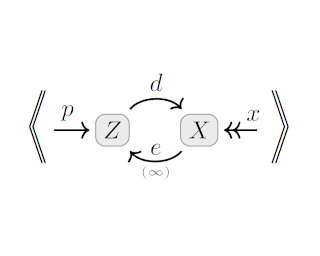In a world blessed with a great diversity of loss functions, we argue that that choice between them is not a matter of taste or pragmatics, but of model. Probabilistic depencency graphs (PDGs) are probabilistic models that come equipped with a measure of "inconsistency". We prove that many standard loss functions arise as the inconsistency of a natural PDG describing the appropriate scenario, and use the same approach to justify a well-known connection between regularizers and priors. We also show that the PDG inconsistency captures a large class of statistical divergences, and detail benefits of thinking of them in this way, including an intuitive visual language for deriving inequalities between them. In variational inference, we find that the ELBO, a somewhat opaque objective for latent variable models, and variants of it arise for free out of uncontroversial modeling assumptions---as do simple graphical proofs of their corresponding bounds. Finally, we observe that inconsistency becomes the log partition function (free energy) in the setting where PDGs are factor graphs.

Alex Nowak · Alessandro Rudi · Francis Bach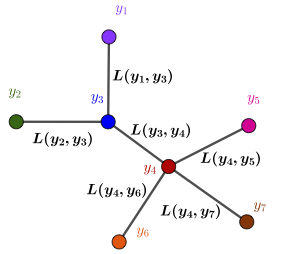The foundational concept of Max-Margin in machine learning is ill-posed for output spaces with more than two labels such as in structured prediction. In this paper, we show that the Max-Margin loss can only be consistent to the classification task under highly restrictive assumptions on the discrete loss measuring the error between outputs. These conditions are satisfied by distances defined in tree graphs, for which we prove consistency, thus being the first losses shown to be consistent for Max-Margin beyond the binary setting. We finally address these limitations by correcting the concept of Max-Margin and introducing the Restricted-Max-Margin, where the maximization of the loss-augmented scores is maintained, but performed over a subset of the original domain. The resulting loss is also a generalization of the binary support vector machine and it is consistent under milder conditions on the discrete loss.

Tin Nguyen · Brian Trippe · Tamara Broderick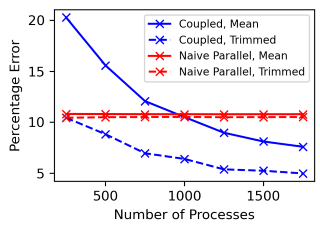Markov chain Monte Carlo (MCMC) methods are often used in clustering since they guarantee asymptotically exact expectations in the infinite-time limit. In finite time, though, slow mixing often leads to poor performance. Modern computing environments offer massive parallelism, but naive implementations of parallel MCMC can exhibit substantial bias. In MCMC samplers of continuous random variables, Markov chain couplings can overcome bias. But these approaches depend crucially on paired chains meetings after a small number of transitions. We show that straightforward applications of existing coupling ideas to discrete clustering variables fail to meet quickly. This failure arises from the "label-switching problem": semantically equivalent cluster relabelings impede fast meeting of coupled chains. We instead consider chains as exploring the space of partitions rather than partitions' (arbitrary) labelings. Using a metric on the partition space, we formulate a practical algorithm using optimal transport couplings. Our theory confirms our method is accurate and efficient. In experiments ranging from clustering of genes or seeds to graph colorings, we show the benefits of our coupling in the highly parallel, time-limited regime.

Quan Zhou · Aaron Smith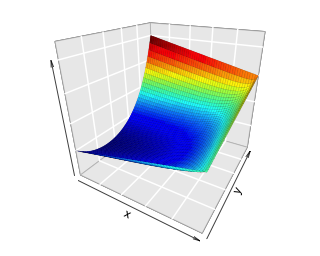Informed Markov chain Monte Carlo (MCMC) methods have been proposed as scalable solutions to Bayesian posterior computation on high-dimensional discrete state spaces, but theoretical results about their convergence behavior in general settings are lacking. In this article, we propose a class of MCMC schemes called informed importance tempering (IIT), which combine importance sampling and informed local proposals, and derive generally applicable spectral gap bounds for IIT estimators. Our theory shows that IIT samplers have remarkable scalability when the target posterior distribution concentrates on a small set. Further, both our theory and numerical experiments demonstrate that the informed proposal should be chosen with caution: the performance may be very sensitive to the shape of the target distribution. We find that the square-root proposal weighting'' scheme tends to perform well in most settings.

Alejandro Catalina · Paul Bürkner · Aki Vehtari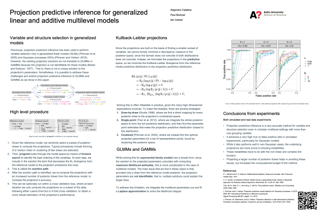Projection predictive inference is a decision theoretic Bayesian approach that decouples model estimation from decision making.Given a reference model previously built including all variables present in the data, projection predictive inference projects its posterior onto a constrained space of a subset of variables. Variable selection is then performed by sequentially adding relevant variables until predictive performance is satisfactory. Previously, projection predictive inference has been demonstrated only for generalized linear models (GLMs) and Gaussian processes (GPs) where it showed superior performance to competing variable selection procedures. In this work, we extend projection predictive inference to support variable and structure selection for generalized linear multilevel models (GLMMs) and generalized additive multilevel models (GAMMs). Our simulative and real-world experiments demonstrate that our method can drastically reduce the model complexity required to reach reference predictive performance and achieve good frequency properties.

Kristy Choi · Chenlin Meng · Yang Song · Stefano Ermon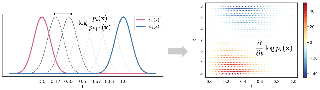Density ratio estimation (DRE) is a fundamental machine learning technique for comparing two probability distributions.However, existing methods struggle in high-dimensional settings, as it is difficult to accurately compare probability distributions based on finite samples.In this work we propose DRE-$\infty$, a divide-and-conquer approach to reduce DRE to a series of easier subproblems. Inspired by Monte Carlo methods, we smoothly interpolate between the two distributions via an infinite continuum of intermediate bridge distributions. We then estimate the instantaneous rate of change of the bridge distributions indexed by time (the time score'')---a quantity defined analogously to data (Stein) scores---with a novel time score matching objective. Crucially, the learned time scores can then be integrated to compute the desired density ratio. In addition, we show that traditional (Stein) scores can be used to obtain integration paths that connect regions of high density in both distributions, improving performance in practice. Empirically, we demonstrate that our approach performs well on downstream tasks such as mutual information estimation and energy-based modeling on complex, high-dimensional datasets.
Ulysse Marteau-Ferey · Francis Bach · Alessandro Rudi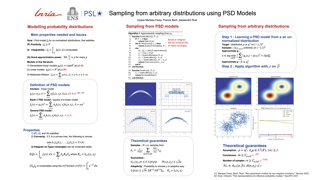In many areas of applied statistics and machine learning, generating an arbitrary number of inde- pendent and identically distributed (i.i.d.) samples from a given distribution is a key task. When the distribution is known only through evaluations of the density, current methods either scale badly with the dimension or require very involved implemen- tations. Instead, we take a two-step approach by first modeling the probability distribution and then sampling from that model. We use the recently introduced class of positive semi-definite (PSD) models which have been shown to be ecient for approximating probability densities. We show that these models can approximate a large class of densities concisely using few evaluations, and present a simple algorithm to eectively sample from these models. We also present preliminary empirical results to illustrate our assertions.

Kirill Neklyudov · Max Welling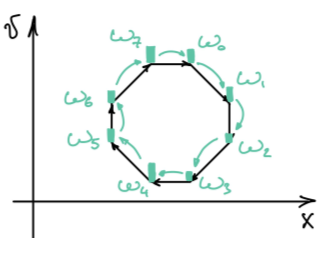Markov Chain Monte Carlo (MCMC) algorithms ubiquitously employ complex deterministic transformations to generate proposal points that are then filtered by the Metropolis-Hastings-Green (MHG) test. However, the condition of the target measure invariance puts restrictions on the design of these transformations. In this paper, we first derive the acceptance test for the stochastic Markov kernel considering arbitrary deterministic maps as proposal generators. When applied to the transformations with orbits of period two (involutions), the test reduces to the MHG test. Based on the derived test we propose two practical algorithms: one operates by constructing periodic orbits from any diffeomorphism, another on contractions of the state space (such as optimization trajectories). Finally, we perform an empirical study demonstrating the practical advantages of both kernels.

Ilias Diakonikolas · Daniel Kane · Pasin Manurangsi · Lisheng Ren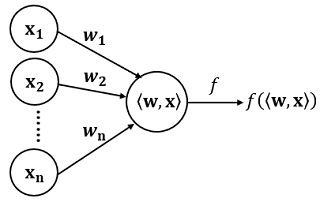We study the problem of distribution-free learning of a single neuronunder adversarial label noise with respect to the squared loss.For a wide range of activation functions, including ReLUs and sigmoids,we prove hardness of learning results in the Statistical Query model andunder a well-studied assumption on the complexity of refuting XOR formulas.Specifically, we establish that no polynomial-time learning algorithm, even improper,can approximate the optimal loss value within any constant factor.

Nicole Mücke · Enrico Reiss · Jonas Rungenhagen · Markus Klein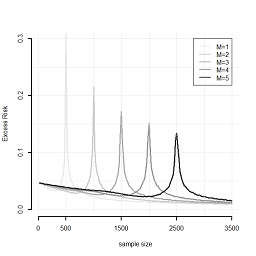While large training datasets generally offer improvement in model performance, thetraining process becomes computationally expensive and time consuming. Distributedlearning is a common strategy to reduce the overall training time by exploiting multiplecomputing devices. Recently, it has been observed in the single machine setting thatoverparameterization is essential for benign overfitting in ridgeless regression in Hilbert spaces. We show that in this regime, data splitting has a regularizing effect, hence improving statistical performance and computational complexity at the same time. We further provide a unified framework that allows to analyze both the finite and infinite dimensional setting. We numerically demonstrate the effect of different model parameters.

Yongchan Kwon · James Zou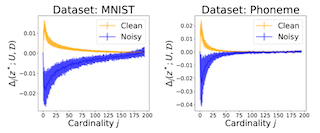Data Shapley has recently been proposed as a principled framework to quantify the contribution of individual datum in machine learning. It can effectively identify helpful or harmful data points for a learning algorithm. In this paper, we propose Beta Shapley, which is a substantial generalization of Data Shapley. Beta Shapley arises naturally by relaxing the efficiency axiom of the Shapley value, which is not critical for machine learning settings. Beta Shapley unifies several popular data valuation methods and includes data Shapley as a special case. Moreover, we prove that Beta Shapley has several desirable statistical properties and propose efficient algorithms to estimate it. We demonstrate that Beta Shapley outperforms state-of-the-art data valuation methods on several downstream ML tasks such as: 1) detecting mislabeled training data; 2) learning with subsamples; and 3) identifying points whose addition or removal have the largest positive or negative impact on the model.

Anant Raj · Pooria Joulani · Andras Gyorgy · Csaba Szepesvari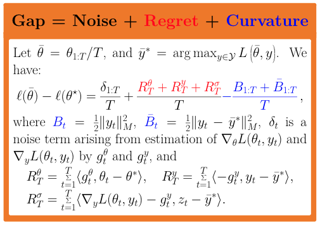Gradient temporal difference (GTD) algorithms are provably convergent policy evaluation methods for off-policy reinforcement learning. Despite much progress, proper tuning of the stochastic approximation methods used to solve the resulting saddle point optimization problem requires the knowledge of several (unknown) problem-dependent parameters. In this paper we apply adaptive step-size tuning strategies to greatly reduce this dependence on prior knowledge, and provide algorithms with adaptive convergence guarantees. In addition, we use the underlying refined analysis technique to obtain new O(1/T) rates that do not depend on the strong-convexity parameter of the problem, and also apply to the Markov noise setting, as well as the unbounded i.i.d. noise setting.

Sharan Vaswani · Olivier Bachem · Simone Totaro · Robert Müller · Shivam Garg · Matthieu Geist · Marlos C. Machado · Pablo Samuel Castro · Nicolas Le Roux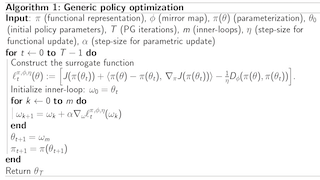Common policy gradient methods rely on the maximization of a sequence of surrogate functions. In recent years, many such surrogate functions have been proposed, most without strong theoretical guarantees, leading to algorithms such as TRPO, PPO, or MPO. Rather than design yet another surrogate function, we instead propose a general framework (FMA-PG) based on functional mirror ascent that gives rise to an entire family of surrogate functions. We construct surrogate functions that enable policy improvement guarantees, a property not shared by most existing surrogate functions. Crucially, these guarantees hold regardless of the choice of policy parameterization. Moreover, a particular instantiation of FMA-PG recovers important implementation heuristics (e.g., using forward vs reverse KL divergence) resulting in a variant of TRPO with additional desirable properties. Via experiments on simple reinforcement learning problems, we evaluate the algorithms instantiated by FMA-PG. The proposed framework also suggests an improved variant of PPO, whose robustness and efficiency we empirically demonstrate on the MuJoCo suite.

Jan Macdonald · Stephan Wäldchen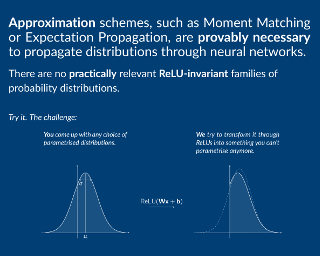We give a complete characterisation of families of probability distributions that are invariant under the action of ReLU neural network layers (in the same way that the family of Gaussian distributions is invariant to affine linear transformations). The need for such families arises during the training of Bayesian networks or the analysis of trained neural networks, e.g., in the context of uncertainty quantification (UQ) or explainable artificial intelligence (XAI).We prove that no invariant parametrised family of distributions can exist unless at least one of the following three restrictions holds: First, the network layers have a width of one, which is unreasonable for practical neural networks. Second, the probability measures in the family have finite support, which basically amounts to sampling distributions. Third, the parametrisation of the family is not locally Lipschitz continuous, which excludes all computationally feasible families.Finally, we show that these restrictions are individually necessary. For each of the three cases we can construct an invariant family exploiting exactly one of the restrictions but not the other two.

Arman Adibi · Aryan Mokhtari · Hamed Hassani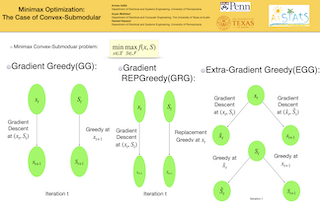Minimax optimization has been central in addressing various applications in machine learning, game theory, and control theory. Prior literature has thus far mainly focused on studying such problems in the continuous domain, e.g., convex-concave minimax optimization is now understood to a significant extent. Nevertheless, minimax problems extend far beyond the continuous domain to mixed continuous-discrete domains or even fully discrete domains. In this paper, we study mixed continuous-discrete minimax problems where the minimization is over a continuous variable belonging to Euclidean space and the maximization is over subsets of a given ground set. We introduce the class of convex-submodular minimax problems, where the objective is convex with respect to the continuous variable and submodular with respect to the discrete variable. Even though such problems appear frequently in machine learning applications, little is known about how to address them from algorithmic and theoretical perspectives. For such problems, we first show that obtaining saddle points are hard up to any approximation, and thus introduce new notions of (near-) optimality. We then provide several algorithmic procedures for solving convex and monotone-submodular minimax problems and characterize their convergence rates, computational complexity, and quality of the final solution according to our notions of optimally. Our …

Jun Ho Yoon · Daniel Jeong · Seyoung Kim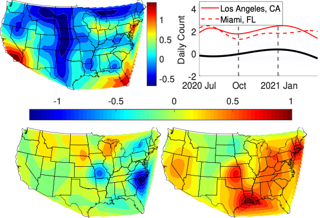We address the multi-task Gaussian process (GP) regression problem with the goal of decomposing input effects on outputs into components shared across or specific to tasks and samples. We propose a family of mixed-effects GPs, including doubly and translated mixed-effects GPs, that performs such a decomposition, while also modeling the complex task relationships. Instead of the tensor product widely used in multi-task GPs, we use the direct sum and Kronecker sum for Cartesian product to combine task and sample covariance functions. With this kernel, the overall input effects on outputs decompose into four components: fixed effects shared across tasks and across samples and random effects specific to each task and to each sample. We describe an efficient stochastic variational inference method for our proposed models that also significantly reduces the cost of inference for the existing mixed-effects GPs. On simulated and real-world data, we demonstrate that our approach provides higher test accuracy and interpretable decomposition.

Hugh Dance · Brooks Paige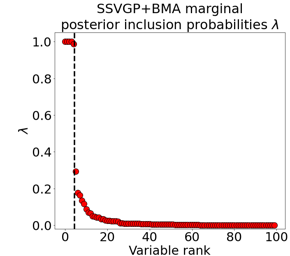Variable selection in Gaussian processes (GPs) is typically undertaken by thresholding the inverse lengthscales of automatic relevance determination kernels, but in high-dimensional datasets this approach can be unreliable. A more probabilistically principled alternative is to use spike and slab priors and infer a posterior probability of variable inclusion. However, existing implementations in GPs are very costly to run in both high-dimensional and large-n datasets, or are only suitable for unsupervised settings with specific kernels. As such, we develop a fast and scalable variational inference algorithm for the spike and slab GP that is tractable with arbitrary differentiable kernels. We improve our algorithm's ability to adapt to the sparsity of relevant variables by Bayesian model averaging over hyperparameters, and achieve substantial speed ups using zero temperature posterior restrictions, dropout pruning and nearest neighbour minibatching. In experiments our method consistently outperforms vanilla and sparse variational GPs whilst retaining similar runtimes (even when n=10^6) and performs competitively with a spike and slab GP using MCMC but runs up to 1000 times faster.

Ningyuan Chen · Xuefeng GAO · Yi Xiong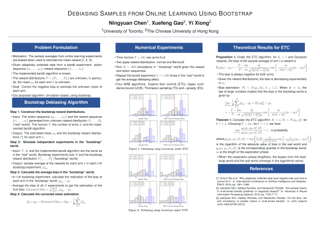It has been recently shown in the literature (Nie et al, 2018; Shin et al, 2019a,b) that the sample averages from online learning experiments are biased when used to estimate the mean reward. To correct the bias, off-policy evaluation methods, including importance sampling and doubly robust estimators, typically calculate the conditional propensity score, which is ill-defined for non-randomized policies such as UCB. This paper provides a procedure to debias the samples using bootstrap, which doesn't require the knowledge of the reward distribution and can be applied to any adaptive policies. Numerical experiments demonstrate the effective bias reduction for samples generated by popular multi-armed bandit algorithms such as Explore-Then-Commit (ETC), UCB, Thompson sampling (TS) and $\epsilon$-greedy (EG). We analyze and provide theoretical justifications for the procedure under the ETC algorithm, including the asymptotic convergence of the bias decay rate in the real and bootstrap worlds.
Lang Liu · Soumik Pal · Zaid Harchaoui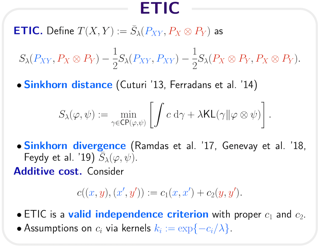We introduce an independence criterion based on entropy regularized optimal transport. Our criterion can be used to test for independence between two samples. We establish non-asymptotic bounds for our test statistic and study its statistical behavior under both the null hypothesis and the alternative hypothesis. The theoretical results involve tools from U-process theory and optimal transport theory. We also offer a random feature type approximation for large-scale problems, as well as a differentiable program implementation for deep learning applications. We present experimental results on existing benchmarks for independence testing, illustrating the interest of the proposed criterion to capture both linear and nonlinear dependencies in synthetic data and real data.

Jie Wang · Rui Gao · Yao Xie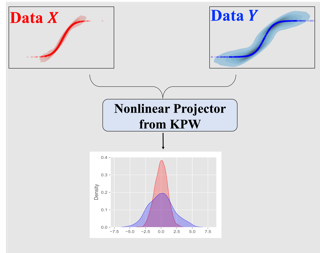We develop a kernel projected Wasserstein distance for the two-sample test, an essential building block in statistics and machine learning: given two sets of samples, to determine whether they are from the same distribution. This method operates by finding the nonlinear mapping in the data space which maximizes the distance between projected distributions. In contrast to existing works about projected Wasserstein distance, the proposed method circumvents the curse of dimensionality more efficiently. We present practical algorithms for computing this distance function together with the non-asymptotic uncertainty quantification of empirical estimates. Numerical examples validate our theoretical results and demonstrate good performance of the proposed method.

Pratik Patil · Alessandro Rinaldo · Ryan Tibshirani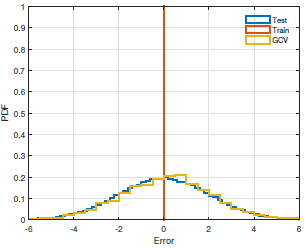We study the problem of estimating the distribution of the out-of-sample prediction error associated with ridge regression. In contrast, the traditional object of study is the uncentered second moment of this distribution (the mean squared prediction error), which can be estimated using cross-validation methods. We show that both generalized and leave-one-out cross-validation (GCV and LOOCV) for ridge regression can be suitably extended to estimate the full error distribution. This is still possible in a high-dimensional setting where the ridge regularization parameter is zero. In an asymptotic framework in which the feature dimension and sample size grow proportionally, we prove that almost surely, with respect to the training data, our estimators (extensions of GCV and LOOCV) converge weakly to the true out-of-sample error distribution. This result requires mild assumptions on the response and feature distributions. We also establish a more general result that allows us to estimate certain functionals of the error distribution, both linear and nonlinear. This yields various applications, including consistent estimation of the quantiles of the out-of-sample error distribution, which gives rise to prediction intervals with asymptotically exact coverage conditional on the training data.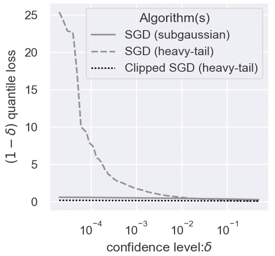We consider the task of heavy-tailed statistical estimation given streaming $p$-dimensional samples. This could also be viewed as stochastic optimization under heavy-tailed distributions, with an additional $O(p)$ space complexity constraint. We design a clipped stochastic gradient descent algorithm and provide an improved analysis, under a more nuanced condition on the noise of the stochastic gradients, which we show is critical when analyzing stochastic optimization problems arising from general statistical estimation problems. Our results guarantee convergence not just in expectation but with exponential concentration, and moreover does so using $O(1)$ batch size. We provide consequences of our results for mean estimation and linear regression. Finally, we provide empirical corroboration of our results and algorithms via synthetic experiments for mean estimation and linear regression.